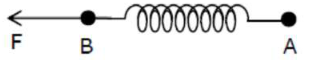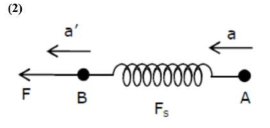# Two masses A and B, each of mass M are fixed together by a massless springs.`
Question:

Two masses $A$ and $B$, each of mass $M$ are fixed together by a massless springs. A force acts on the mass $\mathrm{B}$ as shown in figure. If the mass $\mathrm{A}$ starts moving away from mass $\mathrm{B}$ with acceleration ' $\mathrm{a}$ ', than the acceleration of mass $\mathrm{B}$ will be:1. $\frac{\mathrm{F}+\mathrm{Ma}}{\mathrm{M}}$

2. $\frac{\mathrm{F}-\mathrm{Ma}}{\mathrm{M}}$

3. $\frac{M a-F}{M}$

4. $\frac{M F}{F+M a}$

Correct Option: , 2

Solution:$F-F_{s}=M a^{\prime}$

$a^{\prime}=\frac{F}{M}-9$

$\frac{F-m a}{M}$